# How to Get the length of a Queue in PythonLast updated: Feb 18, 2023
5 min## #Get the length of a Queue in Python

To get the length of a queue in Python:

1. Use the `len()` function to get the length of a deque object.
2. Use the `qsize()` method to get the length of a queue object.
main.py
```Copied!```from collections import deque
import queue

deq = deque(['a', 'b', 'c'])

# ✅ get length of deque object
print(len(deq))  # 👉️ 3

q = queue.Queue()

for item in range(15):
q.put(item)

# ✅ get length of queue object
print('size of queue: ', q.qsize()) # 👉️ 15
``````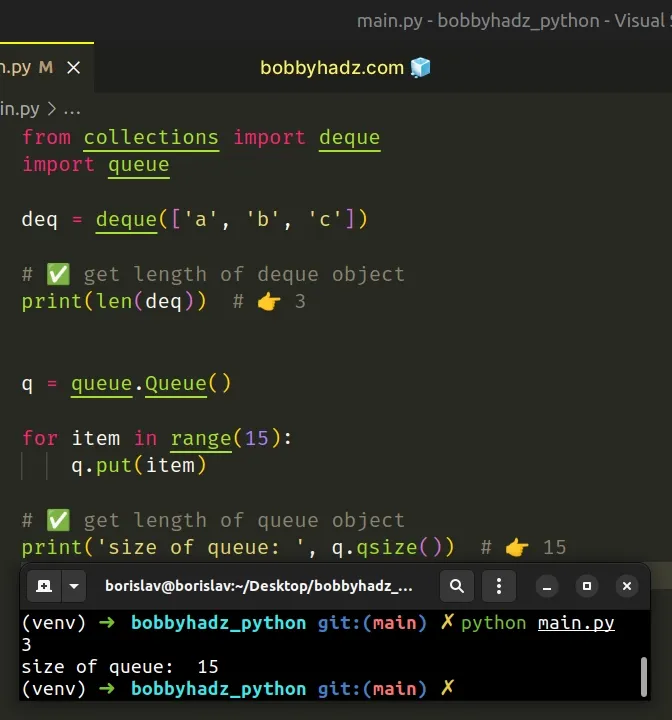We used the `len()` function to get the length of a deque object.

The len() function returns the length (the number of items) of an object.

main.py
```Copied!```from collections import deque

deq = deque(['a', 'b', 'c', 'd'])

# 👇️ get length of deque object
print(len(deq))  # 👉️ 4
``````

The argument the function takes may be a sequence (a string, tuple, list, range or bytes) or a collection (a dictionary, set, or frozen set).

The `len()` function is always guaranteed to return the correct length of the `deque` object, regardless if you add or remove items.

main.py
```Copied!```from collections import deque

deq = deque(['a', 'b'])

deq.append('c')
deq.append('d')

print(len(deq))  # 👉️ 4

deq.remove('d')

print(len(deq))  # 👉️ 3
``````

If you use the `queue` module, use the `qsize()` method to get the length of the queue.

main.py
```Copied!```import queue

q = queue.Queue()

for item in range(15):
q.put(item)

# 👇️ get the length of a queue object
print('size of queue: ', q.qsize()) # 👉️ 15

# 👇️ check if queue is empty
print(q.empty())  # 👉️ False
``````

The Queue.qsize method returns the approximate size of the queue.

You can use the Queue.empty method to check if the queue is empty.

## #Convert a List to a Queue in Python

You can use the `collections.deque` class to convert a list to a queue in Python.

main.py
```Copied!```from collections import deque

my_list = [1, 2, 3]

# ✅ convert list to queue
deq = deque(my_list)

print(deq)  # 👉️ deque([1, 2, 3])

deq.append(4)

print(deq)  # 👉️ deque([1, 2, 3, 4])

print(deq.pop())  # 👉️ 4

print(deq.popleft())  # 👉️ 1
``````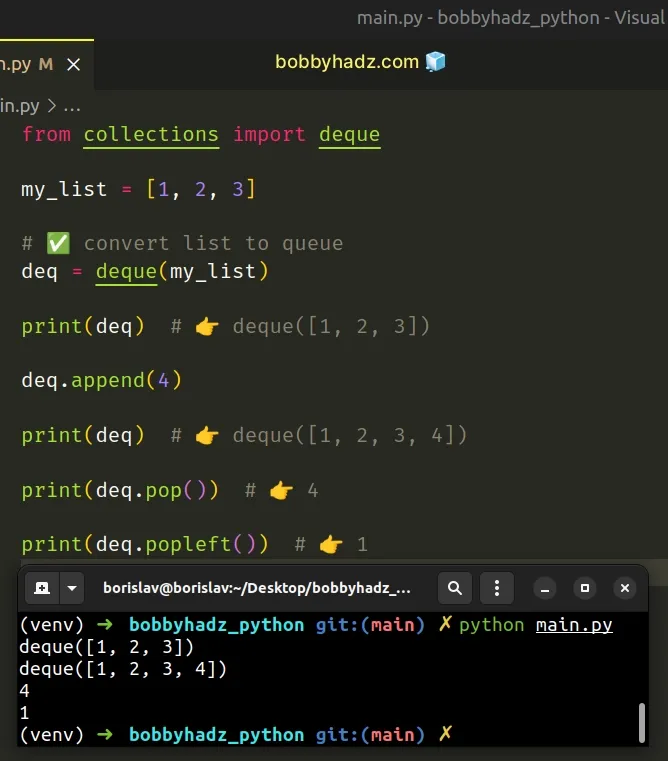The collections.deque class takes an iterable and initializes a `deque` object (a double-ended queue).

The class has atomic `append()`, implements the `popleft()` method and supports indexing and membership testing.

## #Use a `for` loop when the queue is created via the `queue` module

If you need to use the `queue` module to create your queue, use a for loop to convert the list to a queue.

main.py
```Copied!```import queue

my_list = [1, 2, 3]

q = queue.Queue()

for item in my_list:
q.put(item)

print(q.queue)  # 👉️ deque([1, 2, 3])
``````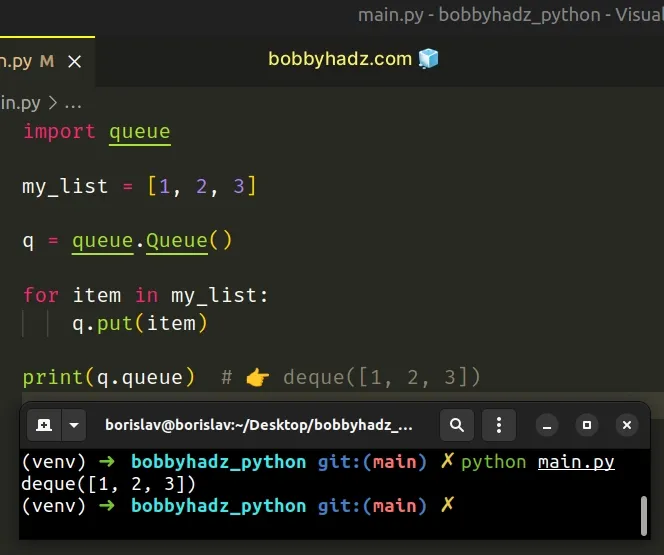The Queue.put method puts an item in the queue.

The `queue` attribute on the queue points to a `deque` object which is much more full-featured.

You should only use the `queue` module if you are running your code in a multi-threaded environment.

Here are some of the most commonly used methods the `deque` class implements.

main.py
```Copied!```from collections import deque

my_list = [1, 2, 3]

deq = deque(my_list)

# 👇️ add a value to the right side of the deque
deq.append(4)

# 👇️ add a value to the left side of the deque
deq.appendleft(0)

# 👇️ extend the right side of the deque by appending the items from the iterable
deq.extend([5, 6])

# 👇️ remove and return an element from the right side of the deque
print(deq.pop())  # 👉️ 6

# 👇️ remove and return an element from the left side of the deque
print(deq.popleft())  # 👉️ 0

print(deq)  # 👉️ deque([1, 2, 3, 4, 5])

deq.clear()

print(deq)  # 👉️ deque([])
``````

## #Create a Fixed-size Queue in Python

You can use the deque class to create a fixed-size queue.

The class takes a `maxlen` argument that determines the maximum length of the deque.

main.py
```Copied!```from collections import deque

deq = deque(maxlen=3)

deq.extend([1, 2, 3])

print(deq)  # 👉️ deque([1, 2, 3], maxlen=3)

deq.append(4)

print(deq)  # 👉️ deque([2, 3, 4], maxlen=3)
``````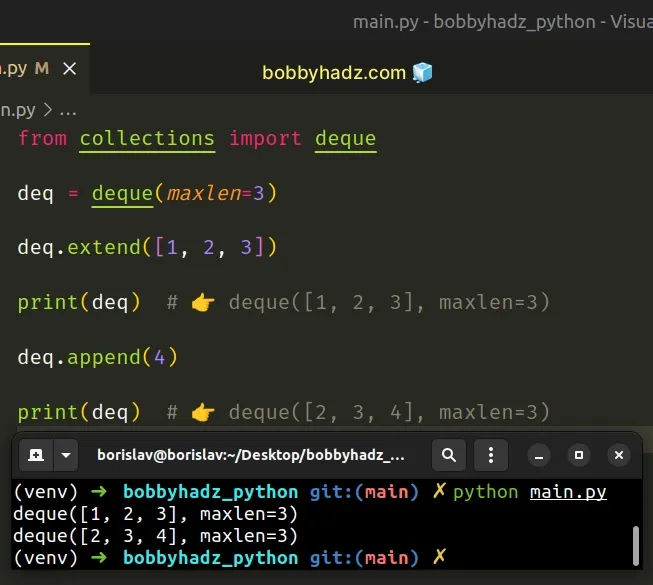We used the collections.deque class to initialize a `deque` object.

You can also pass an iterable to the class to initialize the `deque` object with items.

main.py
```Copied!```from collections import deque

# 👇️ initialize deque with items
deq = deque([1, 2, 3],  maxlen=3)

print(deq)  # 👉️ deque([1, 2, 3], maxlen=3)

deq.append(4)

print(deq)  # 👉️ deque([2, 3, 4], maxlen=3)
``````

The `deque` class takes a `maxlen` argument. If the argument is not provided or is `None`, the `deque` object can grow to an arbitrary length.

If the `maxlen` argument is provided and the `deque` is full, when new items are added, the same number of items are discarded from the opposite end.

If we append a new item to the `deque`, an item from the start of the `deque` is discarded.

main.py
```Copied!```from collections import deque

deq = deque([1, 2, 3],  maxlen=3)

print(deq)  # 👉️ deque([1, 2, 3], maxlen=3)

deq.append(4)

print(deq)  # 👉️ deque([2, 3, 4], maxlen=3)
``````

Conversely, if we use the appendleft method to add an item to the left side of the `deque`, an item from the end of the `deque` is discarded.

main.py
```Copied!```from collections import deque

deq = deque([1, 2, 3],  maxlen=3)

print(deq)  # 👉️ deque([1, 2, 3], maxlen=3)

deq.appendleft(0)

print(deq)  # 👉️ deque([0, 1, 2], maxlen=3)
``````

## #Clear all items from a Queue in Python

If you need to clear all items from a queue in Python:

1. Use the `queue` attribute on the queue to get a `deque` object.
2. Call the `clear()` method on the `deque` object.
3. The `clear` method will remove all elements from the queue.
main.py
```Copied!```import queue

q = queue.Queue()

for item in range(10):
q.put(item)

print(q.queue)  # 👉️ deque([0, 1, 2, 3, 4, 5, 6, 7, 8, 9])

q.queue.clear()

print(q.queue)  # 👉️ deque([])
``````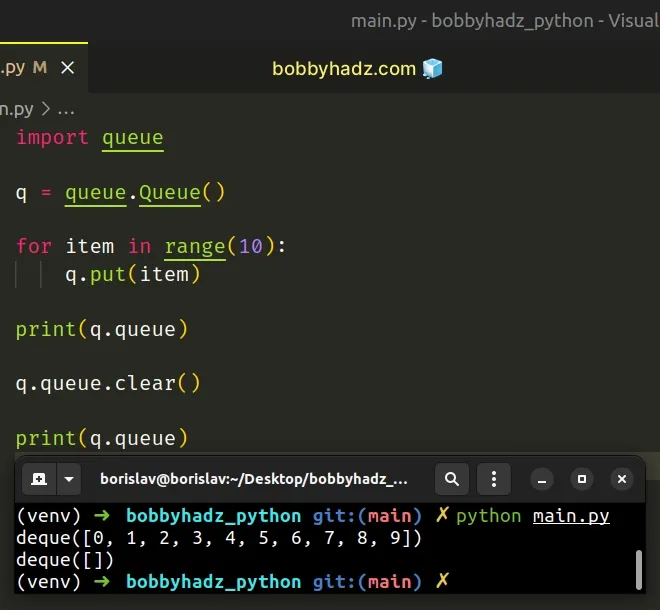The `queue` attribute points to a `deque` object, and `deque` objects implement a clear method.

The `clear()` method removes all elements from the deque and leaves it with a length of `0`.

If you need to make the operation thread-safe, use a `mutex` lock.

main.py
```Copied!```import queue

q = queue.Queue()

for item in range(10):
q.put(item)

print(q.queue)  # 👉️ deque([0, 1, 2, 3, 4, 5, 6, 7, 8, 9])

# 👇️ use mutex lock
with q.mutex:
q.queue.clear()

print(q.queue)  # 👉️ deque([])
``````

You can use a `mutex` lock if your code runs in a multithreaded environment.

An alternative is to simply create a new queue and delete the old one.

main.py
```Copied!```import queue

q = queue.Queue()

for item in range(10):
q.put(item)

del q  # 👈️ delete old queue

new_q = queue.Queue()

print(new_q.queue)  # 👉️ deque([])
``````